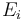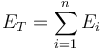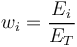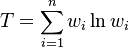Theil Index

Definition

For the purpose of measuring inequality or concentration, the Theil Index (also entropy index) is defined as the sum product of relative values of the distribution, times the natural logarithm of the same values.

Details

More precisely, if we have n values$E_i$ summing up to a total value of$E_T = \sum^{n}_{i=1} E_{i}$

where each fraction is defined as$w_{i} = \frac{E_i}{E_T}$

then the Theil index is defined as$T = \sum^{n}_{i=1} w_{i} \ln{w_{i}}$

None

None

None

Implementations

Open Source implementations of the Theil index are available in